Latest SSC jobs   »   SSC Calendar 2022   »   Complementary Angles

# Complementary Angles – Definition, Formula, Trigonometry and Examples

## Complementary Angles

Complementary Angles: When two angles are added and the sum of values is 90° called complementary angles. And both the angles are called complement each other.  In geometry, if the sum of any two angles measures equal to the right angle and such pairs of angles are termed complementary angles. The values of pair of angles followed by 60° and 30°, 40° and 50°, etc. whose sum of values will be 90°.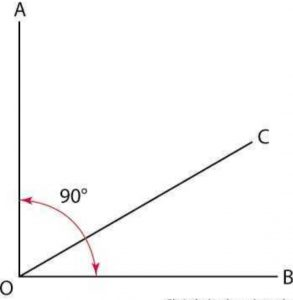Here the angles of AOC and BOC are complementary.

## What are Complementary Angles?

The complementary angles are identified by only the values of angles. The values of angles should be equal to the right angle of 90°. If the values of the sum of two angles are equal to the right angle known by complementary angles. The angle whose sum of values is equal to 90° then the pair of angles are termed as complementary angles. You can see the complementary angles in the above figure in which two angles form a right angle.

## Complementary Angles Definition

The complementary angles are defined as the pair of two angles whose sum of values is equal to the 90°. The values of both the angles form a right angle of 90°.

Two angles are said to be complementary angles if their sum is equal to 90°. It is a very essential criterion for complementary angles. In the above figure the angles AOC and BOC having values in order of 30°&60°, 40°&50°, 65°&25°, 48°&42°, and so on their sum will be 90°. The angles which form a pair of complementary angles are called complements to each other.

## Two Types of Complementary Angles

As we discussed when any two angles having their sum of values form a right angle are called complementary angles. The angles firms a pair of complementary angles are called complement to each other. There are the following two types of complementary angles which are discussed in detail.

The pair of angles having a common arm and vertex are called adjacent complementary angles. The figure is shown below in which angles AOC and BOC have the same arm and vertex which are adjacent complementary angles.The pair of two complementary angles which are not adjacent to each other are called non-adjacent complementary angles. It has no common arm and vertex but the sum of both angles should be 90° always. The non-adjacent complementary angles are shown in the figure below.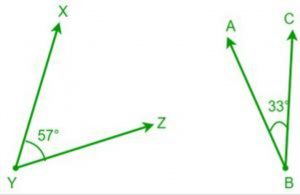In the above figure angles, XYZ and ABC are complementary angles as their sum is equal to 90°.

## Complementary Angles and Supplementary Angles

The identification of complementary and supplementary angles is done based on the addition of two angles. If the addition of two angles gives 90° then it is called complementary angles and if the values of the sum of two angles will be 180° then it is called supplementary angles. The basis of all these angles is angle measurement. The pair of angles that forms supplementary angles whose values equal to 180° are called supplements to each other. On joining all the two supplementary angles we will get a straight line. The complementary angles and supplementary angles can be understood through the below figure.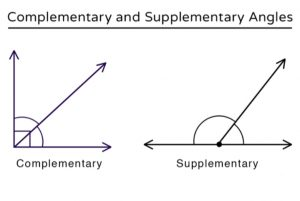The difference between both complementary angles and supplementary angles can be observed in the above figure. Generally, they are both opposite to each other.

## Complementary Angles Examples

The complementary angles are the angles whose sum of values gives a right angle i.e. 90°. The pairs of complementary angles whose values get equal to 90° are complements of each other. The complementary angles can be in the order of 30°&60°, 40°&50°, 55°&35°, and so on. The examples of complementary angles are shown below.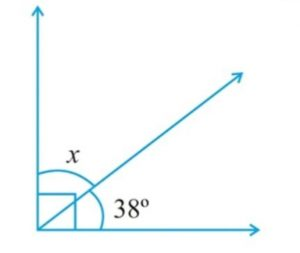In the above figure, one angle is given 38° and another angle is missing we need to find the value of angle x

We know that the sum of two complementary angles is 90°

then, x + 38° = 90°, x = 52°

The value of angle x is 52°.

You can find the values of other angles also from using the properties of angles.

## Complementary Angles in Trigonometry

In trigonometry, there are many applications of complementary angles. The trigonometric ratios can be found by using complementary angles.

Consider a right angled triangle ABC, in which B is right angle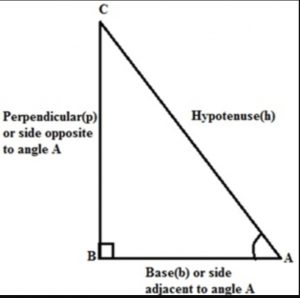∠A and ∠C form a complementary pair.

⇒ ∠A + ∠C = 90°

The relationship between the acute angle and side having longer length can be found using right-angle triangle is expressed by trigonometric ratios. For the given right angle triangle, the  trigonometric ratios of ∠A is given as follows:

sin A = BC/AC

cos A = AB/AC

tan A =BC/AB

csc A = 1/sin A = AC/BC

sec A =1/cos A = AC/AB

cot A = 1/tan A = AB/BC

The trigonometric ratio of the complement of ∠A. It means that the ∠C can be given as 90° – ∠A

As ∠C = 90°- A, and the side opposite to 90° – A is AB and the side adjacent to the angle 90°- A is BC as shown in the figure shown above.

sin (90°- A) = AB/AC

cos (90°- A) = BC/AC

tan (90°- A) = AB/BC

csc (90°- A) =1/sin (90°- A) = AC/AB

sec (90°- A) = 1/cos (90°- A) = AC/BC

cot (90°- A) = 1/tan (90°- A)  = BC/AB

Comparing the above set of trigonometric ratios following relations can also be determined:

sin (90°- A) = cos A ; cos (90°- A) = sin A

tan (90°- A) = cot A; cot (90°- A) = tan A

sec (90°- A) = csc A; CSC (90°- A) = sec A

These relations are valid for all the values of A that lie between 0° and 90°.

## Complementary Angles: Solved Examples

1. Find the complement of the angle 2/3 of 90°.

Solution: 2/3 of 90° = 60°

Complement of 60° = 90° – 60° = 30°

Therefore, the complement of the angle 2/3 of 90° = 30°

2. The values of two complementary angles are (2x – 7)° and (x + 4)°. Find the value of x.

Solution: According to the problem, (2x – 7)° and (x + 4)°, are complementary angles’ so we get;

(2x – 7)° + (x + 4)° = 90°

or, 2x – 7° + x + 4° = 90°

or, 2x + x – 7° + 4° = 90°

or, 3x – 3° = 90°

or, 3x – 3° + 3° = 90° + 3°

or, 3x = 93°

or, x = 93°/3°

or, x = 31°

Therefore, the value of x = 31°.

3. Find the supplement of the angle 4/5 of 90°.

Solution: 4/5 of 90°

4/5 × 90° = 72°

Supplement of 72° = 180° – 72° = 108°

Therefore, as a supplement the angle 4/5 of 90° = 108°

4. The values of two supplementary angles are (3x + 15)° and (2x + 5)°. Find the value of x.

Solution: According to the given problem, (3x + 15)° and (2x + 5)°, are complementary angles’ so we get;

(3x + 15)° + (2x + 5)° = 180°

or, 3x + 15° + 2x + 5° = 180°

or, 3x + 2x + 15° + 5° = 180°

or, 5x + 20° = 180°

or, 5x + 20° – 20° = 180° – 20°

or, 5x = 160°

or, x = 160°/5°

or, x = 32°

Therefore, the value of x = 32°.

5. If one complementary angle is 49° and the other complementary angle is x° then find the value of angle x.

Solution: We know that the sum of two complementary angles ais90°

we have 49° + x = 90°

then x = 90° – 49°

x = 41°

6. If one of the supplementary angles measures 32° while the other is angle P° then find the value of angle P.

Solution: We know that the sum of two supplementary angles is equal to 180°

then according to the given problem       32° + P° = 180°

P° = 180° – 32°

P° = 148°

7. The difference between the two given complementary angles is 180°. Find the measure of the angle.

Solution: Let one angle be of measure x°.

Then complement of x° = (90 – x)

Difference = 18°

Therefore, (90° – x) – x = 18°

or, 90° – 2x = 18°

or, 90° – 90° – 2x = 18° – 90°

or, -2x = -72°

or, x = 72°/2°

or, x = 36°

Also, 90° – x

= 90° – 36°

= 54°.

Therefore, the two angles are 36°, and 54°

8. POQ is a straight line and OS stands on PQ. Find the value of x and the measure of ∠ POS, ∠ SOR, and ∠ ROQ.

Solution:

POQ is a straight line.

Therefore, ∠POS + ∠SOR + ∠ROQ = 180°

(5x + 4°) + (x – 2°) + (3x + 7°) = 180°

or, 5x + 4° + x – 2° + 3x + 7° = 180°

or, 5x + x + 3x + 4° – 2° + 7° = 180°

or, 9x + 9° = 180°

or, 9x + 9° – 9° = 180° – 9°

or, 9x = 171°

or, x = 171/9

or, x = 19°

Put the value of x = 19°

Therefore, x – 2

= 19 – 2

= 17°

Again, 3x + 7

= 3 × 19° + 7°

= 570 + 7°

= 64°

And again, 5x + 4

= 5 × 19° + 4°

= 95° + 4°

= 99°

Therefore, the measure of the three angles is 17°, 64°, and 99°.

9. The measure of an angle is 3/4 of 60°. What is the measure of the complementary angle?

Solution: Let x be the measure of a complementary angle required.

Given: The measure of an angle is 3/4 of 60°

3/4 ⋅ 60°  =  45°

Since x and 45° are complementary angles, we have

x + 45  =  90

Subtract 45° from each side.

x  =  45

So, the measure of the complementary angle is 45°.

10. Two angles are supplementary. If one angle is 36° less than two of the other angle, find the two angles.

Solution: Consider x and y to the two angle that are supplementary.

x + y  =  180° —–>(1)

As per the question “one angle is 36

Given that One angle is 36° less than twice the other angle.

Then,

x  =  2y – 36 —–>(2)

Now substitute (2y – 6) for x in (1),

(1)—–> 2y – 36 + y  =  180

3y – 36  =  180

3y  =  216

Divide each side by 3.

y  =  72

Now, Substitute 72 for y in (2).

(2)—–> x  =  2(72) – 36

x  =  144 – 36

x  =  108

So, the two angles are 108° and 72°.

### Complementary Angles: FAQs

Que.1 What are the complementary angles?

Ans – The pairs of angles having a sum of angles is 90° are called complementary angles and both the angles are called complements to each other.

Que.2 Define Supplementary angles?

Ans – The angles having their sum of angles will be 180° called supplementary angles. Both the angles forming these angles are called supplementary angles.

Que.3 What are alternate and non-alternate complementary angles?

Ans – The angles having a common arm and vertex are called adjacent complementary angles while the angles having different vertex are called non-alternate complementary angles.

Que.4 What is the sum of complementary angles and supplementary angles?

Ans – The sum of complementary angles is 90° and the sum of supplementary angles is 180°.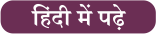#### Congratulations!General Awareness & Science Capsule PDF